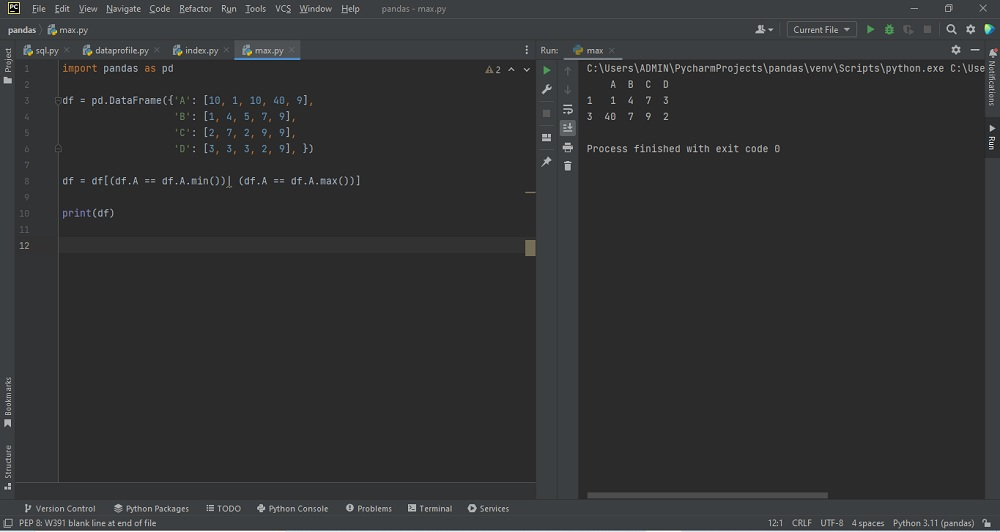# Selecting rows with min and max values in pandasby vsasikalabeUpdated: Feb 28, 2023Solution Kit

We have to create a data frame for selecting the minimum and maximum values from the data frame. We can use df. loc[df[col]. idxmax()] or (df = df[(df.A==df.A.min())|(df.A==df.A.max())] to find the maximum column value and to return its corresponding row values in Pandas. To return a Series with the minimum value of each column, the min() method is used. The max() method searches column-wise and returns the minimum value for each row by specifying the column axis ( axis='columns' ). Sometimes it is also useful to calculate the range of a dataset with the min and max values.

Steps

• Create a two-dimensional, size-mutable tabular data referred to as df.
• Print the input Data Frame using print(df).
• Initialize a variable(value) for all col and rows to find the maximum value of that column.
• We can find the maximum value to its corresponding row using df.loc[df[col].idxmax()]
• Print the Step 4 output.

To get the minimum value in a data frame row, call the min() function. Call the data frame with the specific column name and min() function to get the minimum value in a particular column.

Syntax:

dataframe['column_name'].min()

Here is an example of how to select rows with minimum and maximum values in Pandas:Preview of the output that you will get on running this code from your IDE.

### Code

In this solution we used pandas library of python.

### Instructions

Follow the steps carefully to get the output easily.

2. Create a new Python file on your IDE.
3. Copy the snippet using the 'copy' and paste it into a Python file.
4. Install pandas on your IDE.
5. Run the file to generate the output.

I hope you found this useful. I have added the link to dependent libraries, and version information in the following sections.

I found this code snippet by searching for ' Selecting rows with min and max values in pandas' in kandi. You can try any such use case!

### Environment Tested

I tested this solution in the following versions. Be mindful of changes when working with other versions.

1. The solution is created in PyCharm 2022.3.
2. The solution is tested on Python 3.11.1
3. Pandas version-1.5.2.

Using this solution, we are able to Select rows with min and max values in pandas with simple steps. This process also facilities an easy way to use, hassle-free method to create a hands-on working version of code which would help us to Select rows with min and max values in pandas.

### Dependent Library

pandasby pandas-dev

Python38689Version:v2.0.2Flexible and powerful data analysis / manipulation library for Python, providing labeled data structures similar to R data.frame objects, statistical functions, and much more

Support
Quality
Security
Reuse

pandasby pandas-dev

Python38689Version:v2.0.2License: Permissive (BSD-3-Clause)

Flexible and powerful data analysis / manipulation library for Python, providing labeled data structures similar to R data.frame objects, statistical functions, and much more
Support
Quality
Security